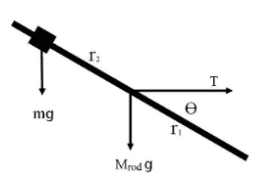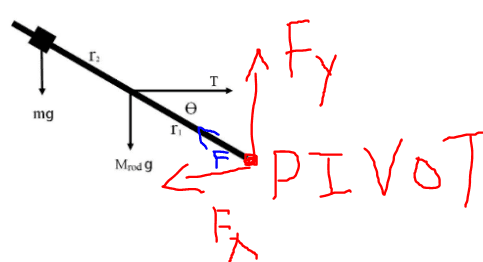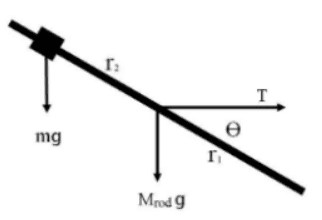# Static Equilibrium of a Rod attached to a Pivot

bbsgirl10
Homework Statement:
Mrod = 0.025, m = 0.075, r1 =0.165m r2 =0.35m, T = 1.21N Angle = 40 degree
What is the formula to find horizontal and vertical components of the force exerted by the pivot on the rod?
1) Equilibrium condition to calculate the horizontal and vertical components of the force exerted by the pivot on the rod.
2) Pivot exerts a force on the rod, why was this not included in the calculation of the torques?
Relevant Equations:
∑ F = 0Homework Helper
Gold Member
At which end of the rod is the pivot?
2) Pivot exerts a force on the rod, why was this not included in the calculation of the torques?

••topsquark and bbsgirl10
bbsgirl10
At which end of the rod is the pivot?

τ1= T r1Sinθ and τ2=( mgr2+Mrodgr1) Cos θHomework Helper
Gold Member
2022 Award
Homework Statement:: Mrod = 0.025, m = 0.075, r1 =0.165m r2 =0.35m, T = 1.21N Angle = 40 degree
We now know where the pivot is meant to be but:
- what are the units for Mrod and m? lbs, kg, grams, metric tons, other?
- is T meant to act horizontally?
- Is r2 the distance between m and the rod's centre, or the distance between m and the pivot?

What is the formula to find horizontal and vertical components of the force exerted by the pivot on the rod?
Since the system (rod & m) is in equilibriun:
vector sum of all x-forces on the system =0
vector sum of all y-forces on the system =0
It's up to you to apply these. Show us your attempt.

2) Pivot exertsa force on the rod, why was this not included in the calculation of the torques?
If a force (F) acts through a point (P), what is the torque of F about P?

••topsquark, bbsgirl10 and Lnewqban
bbsgirl10
We now know where the pivot is meant to be but:
- what are the units for Mrod and m? kg
- is T meant to act horizontally?
T is a string tension
- Is r2 the distance between m and the rod's centre, or the distance between m and the pivot?
Distance from pivot to center hole (r1)
Distance from pivot to brass mass (r2)

Since the system (rod & m) is in equilibriun:
vector sum of all x-forces on the system =0
vector sum of all y-forces on the system =0
It's up to you to apply these. Show us your attempt.
i just want to know the formula to find horizontal and vertical components of the force exerted by the pivot on the rod?
i know the sum of the force will be zero but I don't get it how to calculate fx and fy
If a force (F) acts through a point (P), what is the torque of F about P?

Homework Helper
i know the sum of the force will be zero but I don't get it how to calculate fx and fy
The sum of all forces in the x direction will be zero. Can you write down an equation from that fact?
The sum of all forces in the y direction will be zero. Can you write down an equation from that fact?

••topsquark and bbsgirl10
bbsgirl10
The sum of all forces in the x direction will be zero. Can you write down an equation from that fact?
The sum of all forces in the y direction will be zero. Can you write down an equation from that fact?
For horizontal equilibrium: ∑𝐹𝑥 = 0 (Summation of horizontal forces equal zero)
T - Fx = 0
Fx = T
where Fx= 𝑟1 cos θ
For vertical equilibrium: ∑𝐹𝑦 = 0
-m(g) - Mrod (g) + Fy = 0
Fy = mg + Mrod (g)

is this correct?

Homework Helper
Gold Member
2022 Award
where Fx= 𝑟1 cos θ
r1 is a distance, not a force.

••topsquark and bbsgirl10
bbsgirl10
r1 is a distance, not a force.
i mean the force acting on Fx Is tension on the string which is why I put r1 cosθ

Homework Helper
Gold Member
2022 Award
i mean the force acting on Fx Is tension on the string which is why I put r1 cosθ
Doesn't help.. r1 is not a force, so the equation makes no sense. A force can only equal another force, not a distance.
I should also have pointed out that this is incorrect:
T - Fx = 0
T is not horizontal, Fx is, so they cannot balance.

••topsquark and bbsgirl10
bbsgirl10
Doesn't help.. r1 is not a force, so the equation makes no sense. A force can only equal another force, not a distance.
I should also have pointed out that this is incorrect:

T is not horizontal, Fx is, so they cannot balance.
Oh i see then what are the forces acting on Fx and Fy ?

Homework Helper
Gold Member
2022 Award
Oh i see then what are the forces acting on Fx and Fy ?
Fx is a horizontal force. It needs to be balanced by a horizontal force, or horizontal component of a force. The gravitational forces have no horizontal component, so that leaves only the horizontal component of …?

•topsquark and bbsgirl10
bbsgirl10
Fx is a horizontal force. It needs to be balanced by a horizontal force, or horizontal component of a force. The gravitational forces have no horizontal component, so that leaves only the horizontal component of …?But why T is not horizontal?

bbsgirl10
Fx is a horizontal force. It needs to be balanced by a horizontal force, or horizontal component of a force. The gravitational forces have no horizontal component, so that leaves only the horizontal component of …?
Tension ?

Last edited:
Gold Member
MHB
View attachment 315343
But why T is not horizontal?
Look at your problem statement. What is ##\theta##?

-Dan

bbsgirl10bbsgirl10
Look at your problem statement. What is ##\theta##?

-Dan
Theta refers to the angle measured by the sensor minus 90 degree which is 40 degree.
I m so lost right now what are the factors involved in Fx and Fy? If the sum is zero do I assume the factors that affect also zero?

Gold Member
MHB
Theta refers to the angle measured by the sensor minus 90 degree which is 40 degree.
I m so lost right now what are the factors involved in Fx and Fy? If the sum is zero do I assume the factors that affect also zero?
What sensor? We are apparently missing information and we can't help you properly until we know what's going on. Can you please post the whole problem statement? Strings, sensors, pivot points, and anything else that you may not have mentioned.

-Dan

•Steve4Physics
bbsgirl10
What sensor? We are apparently missing information and we can't help you properly until we know what's going on. Can you please post the whole problem statement? Strings, sensors, pivot points, and anything else that you may not have mentioned.

-Dan

Mrod = 0.025kg, m = 0.075kg, r1 =0.165m r2 =0.35m, T (String Tension) = 1.21N, Angle Theta = 40 degree.
Distance from pivot to center hole (r1)
Distance from pivot to brass mass (r2)
1) Equilibrium condition to calculate the horizontal and vertical components of the force exerted by the pivot on the rod.

Homework Helper
Gold Member
2022 Award
θ is the angle between the rod and T. Although the diagram makes it look like T is horizontal, this will only be true if the angle between the rod and the horizontal is also θ (=40º). This is not specified.

Let α be the angle between the rod and horizontal. We can calculate α by taking moments about the pivot using the given data.

When I calculate α I get α = 64.5º. Not 40º.

So T is not horizontal. But maybe someone else would like to check.

EDIT: Changed in the light of the extra information in Post #19.

•topsquark
Homework Helper
Gold Member
2022 Award
Tension ?
Yes. So if the tension is T, what is its horizontal component?

•topsquark
Homework Helper
Gold Member
θ is the angle between the rod and T. Although the diagram makes it look like T is horizontal, this will only be true if the angle between the rod and the horizontal is also θ (=40º). This is not specified.

Let α be the angle between the rod and horizontal. We can calculate α by taking moments about the pivot using the given data.

When I calculate α I get α = 64.5º. Not 40º.

So T is not horizontal. But maybe someone else would like to check.

EDIT: Changed in the light of the extra information in Post #19.
I got α = 64.5º for the angle between the rod and the horizontal under the assumption that the tension is horizontal and has magnitude 1.21 N.

•topsquark
Homework Helper
Gold Member
2022 Award
I got α = 64.5º for the angle between the rod and the horizontal under the assumption that the tension is horizontal and has magnitude 1.21 N.
The problem with that is ##\alpha= 64.5^o## and ##\theta = 40^o## are not consistent with T being horizontal.

The clockwise moment produced by the tension is ##T r_1 \sin(40^o)## but this doesn’t rely on T being horizontal.

•topsquark
Homework Helper
Gold Member
The problem with that is ##\alpha= 64.5^o## and ##\theta = 40^o## are not consistent with T being horizontal.

The clockwise moment produced by the tension is ##T r_1 \sin(40^o)## but this doesn’t rely on T being horizontal.
The 40° angle is ignored in my calculation. I thought that's what you did when you got the 64.5°. We don't have a precise statement of the problem including this (presumably measured) 40° angle. All we have is
Theta refers to the angle measured by the sensor minus 90 degree which is 40 degree.
If it is the angle between the tension vector and the rod as shown in the drawing, then the angle between between the rod and the horizontal (by my calculation) is 72.2°. I don't feel confident that I understand what's what with this problem.

•topsquark and Lnewqban
Homework Helper
Gold Member
2022 Award
The 40° angle is ignored in my calculation. I thought that's what you did when you got the 64.5°. We don't have a precise statement of the problem including this (presumably measured) 40° angle. All we have is

If it is the angle between the tension vector and the rod as shown in the drawing, then the angle between between the rod and the horizontal (by my calculation) is 72.2°. I don't feel confident that I understand what's what with this problem.
Yes, the problem is very badly posed. We can:
- assume that T is horizontal and not use θ=40° or
- assume that θ=40º and treat T as non-horizontal.
Unless the OP can explain, the problem won't get resolved.

•topsquark, Lnewqban and kuruman